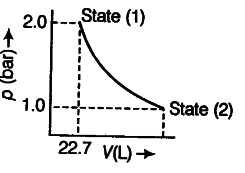# 1.0 mole of a monoatomic ideal gas is expanded from state

1.0 mole of a monoatomic ideal gas is expanded from state (1) to state (2) as shown in a figure. Calculate the work done for the expansion of gas from state (1) to state (2) at 298 K.The given diagram represents that the process is carried out in infinite steps, hence, it is isothermal reversible expansion of the ideal gas from pressure 2.0 atm to 1.0 atm at 298 K.
W = - 2.303 nRT log V2/V1
W = -2.303 x 1 mol x 8.314 J/K/mol x 298 log2
W = -1717.46J